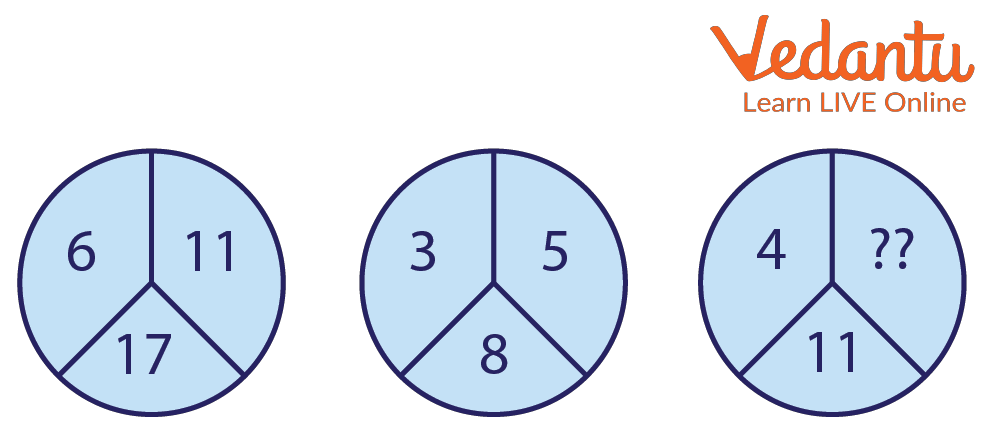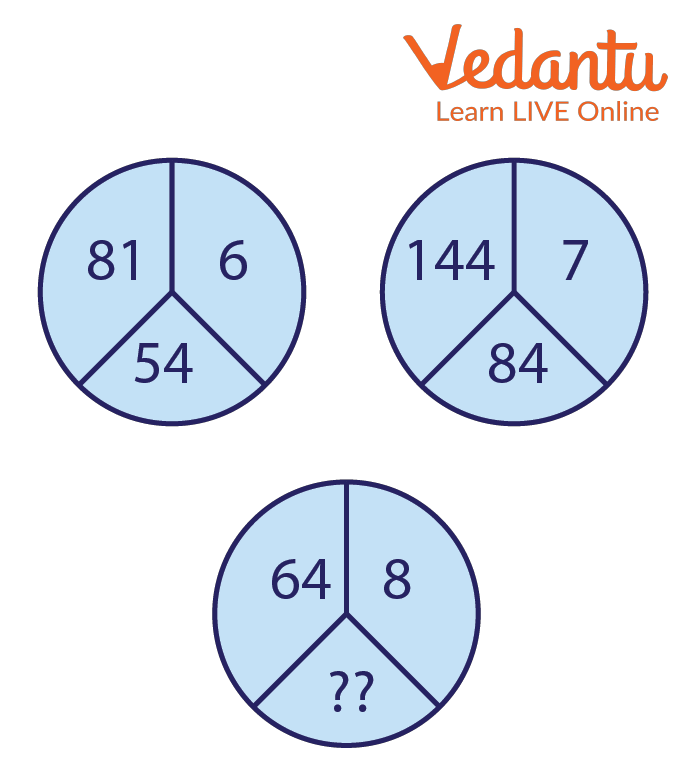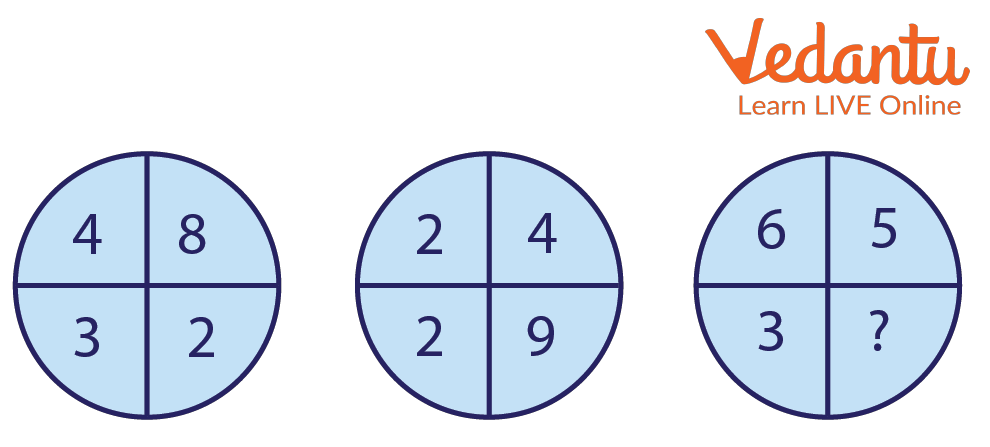Courses
Courses for Kids
Free study material
Free LIVE classes
More

# Circle Puzzle Missing Numbers with AnswersLIVE
Join Vedantu’s FREE Mastercalss

Circle puzzles or pattern of circle are simple brain teasers that can be found everywhere, from newspapers to quiz books. They are popular, especially among children but adults also love to solve these puzzles. Solving these puzzles requires no knowledge, but sometimes they require arithmetic knowledge and strong logical skills. If you enjoy solving circle puzzles or have been wanting to attempt one for a long time, go through this article for a comprehensive guide.

## What is a Circle Puzzle?

A Circle puzzle is a game or issue or numerical question that puts one's creativity or knowledge to the test. In order to get the right or enjoyable answer, the solver of a puzzle is supposed to logically arrange the parts together. Crossword puzzles, word searches, number puzzles, relational puzzles, and logic puzzles are only a few examples of the various puzzle subgenres. Enigmatology is the term for the study of puzzles in academia.

## What Do You Need to Know Before Attempting?

This is the most basic question asked while attempting brain teasers. You do not need any kind of hard maths concept or magical spells, you can solve these puzzles with just a little logic. Recognise a pattern and follow it to find the missing number. Let’s see the procedure of solving puzzles and see the circle puzzles with solution.

## How to Solve a Missing Number Puzzle?

Here are the steps to solve a missing number puzzle.

1. First, recognise a pattern between the numbers of the first set.

2. Verify the pattern for the second set.

3. If the second set satisfies the pattern we got our required pattern, otherwise we look for a different pattern.

4. Once the required pattern is found, use the pattern on the last set to find the missing number.

Now that we have learnt the steps to solve these puzzles, let’s solve a puzzle to get our hands on this. For this purpose, we are going to solve the puzzle given in the image below.Solve the Following Puzzle

To solve the above circular number puzzle, we will follow the steps carefully.

1. The first step is to find a pattern in the first set. Fortunately, we see that the sum of two numbers at the top equals the third number, i.e, $6 + 11 = 17$.

2. Next, we verify the pattern found for the second set of numbers. Here, We see that it works for the second image as $3 + 5 = 8$.

3. Since it works for both images, we can use it for the last one to find the missing number.

4. Hence, applying the pattern for the third image gives us, $4 + {\rm{x}} = 11$. Thus, ${\rm{x}} = 7$.

Great! We have understood how to solve these types of puzzles where we recognise a pattern between numbers and ding the missing number at last. Now, we look at some solved examples to get a better understanding of what we learnt.

## Solved Examples

1. Find the missing number.Find the Missing Number

We see that the square root of 81 is 9. And the product of 9 and 6 gives 54. So we have found a pattern that works for the first image. Let’s verify it for the second image. The square root of 144 is 12 and the product of 12 and 7 is 84. Hence, our pattern satisfies both images, so we can use it on the third image to find the missing number. The square root of 64 is 8 and the product of 8 and 8 is 64. Hence, the missing number should be 64.

2. Find the missing number in the pattern.Find the Missing Number

We see that the sum of all the numbers in the first image is 17 as $4 + 8 + 3 + 2 = 17$. Now we verify this pattern for the second image, $2 + 4 + 2 + 9 = 17$. Hence, the pattern satisfies both images. Thus, we can apply this pattern to find the missing number in the last image, $6 + 5 + 3 + {\rm{x}} = 17$, this gives ${\rm{x}} = 3$.

3. Find the next number in the sequences 0, 2, 2, 4, 5, 9, 14, 23…

Here we see that every term is obtained by adding the previous two terms. Hence the missing number is $23 + 14 = 37$.

4. Find the missing value (5,2,6), (4,3,2), (8,4,?)...

We see that the third term is twice the difference between the first two-term in each triplet. $2 \times [5 - 2] = 6$$2 \times [5 - 2] = 6$, $2 \times [4 - 3] - 2$ hence the missing number is $2 \times [8 - 4] = 8$.

## Conclusion

Circle puzzles with missing numbers are a very interesting brain teaser. They are very fun to play with friends. We have learnt how to solve these puzzles as well as solving some examples. They increase our ability to think logically and increase our arithmetic skills. They can be easily found everywhere, from newspapers to the reasoning section of any competitive examinations.

Last updated date: 03rd Oct 2023
Total views: 84k
Views today: 1.84k

## FAQs on Circle Puzzle Missing Numbers with Answers

1. Why are circle puzzles with missing numbers so common?

Circle puzzles with missing numbers can be very interesting puzzles. They require no prior knowledge to be solved. You can use simple arithmetic and logic to solve these puzzles. They sometimes require strong thinking and they are also not so time-consuming. Hence, they are good puzzle questions for aptitude exams.

2. How do you find the pattern?

We use simple arithmetic to find a relation between the numbers. Then check this relation with the next image to verify.

3. Are circle puzzles with missing numbers hard?

Circle puzzles range from easy to very hard. The easy ones require only simple arithmetic while the hard ones require complex calculations.